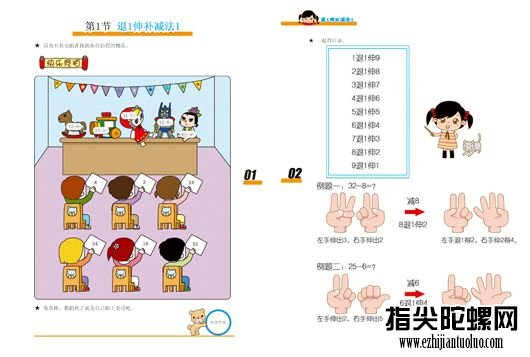两位数乘法速算技巧

原理：设两位数分别为10A+B，10C+D,其积为S,根据多项式展开：

S=(10A+B)×(10C+D)=10A×10C+B×10C+10A×D+B×D，而所谓速算，就是根据其中一些相等或互补(相加为十)的关系简化上式，从而快速得出结果。

A.乘法速算

一.前数相同的：

1.1.十位是1,个位互补,即A=C=1,B+D=10,S=(10+B+D)×10+B×D

方法：百位为二，个位相乘，得数为后积，满十前一。

例：13×17

13+7=2--(“-”在不熟练的时候作为助记符，熟练后就可以不使用了)

3×7=21

-----------------------

221

即13×17=221

1.2.十位是1,个位不互补,即A=C=1,B+D≠10,S=(10+B+D)×10+A×B

方法：乘数的个位与被乘数相加，得数为前积，两数的个位相乘，得数为后积，满十前一。

例：15×17

15+7=22-(“-”在不熟练的时候作为助记符，熟练后就可以不使用了)

5×7=35

-----------------------

255

即15×17=255

1.3.十位相同,个位互补,即A=C,B+D=10,S=A×(A+1)×10+B×D

方法:十位数加1，得出的和与十位数相乘，得数为前积，个位数相乘，得数为后积

例：56×54

(5+1)×5=30--

6×4=24

----------------------

3024

1.4.十位相同,个位不互补,即A=C,B+D≠10,S=A×(A+1)×10+A×B

方法:先头加一再乘头两，得数为前积，尾乘尾，的数为后积，乘数相加，看比十大几或小几，大几就加几个乘数的头乘十，反之亦然

例：67×64

(6+1)×6=42

7×4=28

7+4=11

11-10=1

4228+60=4288

----------------------

4288

方法2：两首位相乘(即求首位的平方)，得数作为前积，两尾数的和与首位相乘，得数作为中积，满十进一，两尾数相乘，得数作为后积。

例：67×64

6×6=36--

(4+7)×6=66-

4×7=28

----------------------

4288

二、后数相同的：

2.1. 个位是1，十位互补即 B=D=1,A+C=10S=10A×10C+101

方法：十位与十位相乘，得数为前积，加上101.。

--8×2=16--

101

-----------------------

1701

2.2.<不是很简便>个位是1，十位不互补即 B=D=1,A+C≠10S=10A×10C+10C+10A+1

方法：十位数乘积，加上十位数之和为前积，个位为1.。

例：71×91

70×90=63--

70+90=16-

1

----------------------

6461

2.3个位是5，十位互补即 B=D=5,A+C=10S=10A×10C+25

方法：十位数乘积，加上十位数之和为前积，加上25。

例：35×75

3×7+5=26--

25

----------------------

2625

2.4<不是很简便>个位是5，十位不互补即 B=D=5,A+C≠10S=10A×10C+525

方法：两首位相乘(即求首位的平方)，得数作为前积，两十位数的和与个位相乘，得数作为中积，满十进一，两尾数相乘，得数作为后积。

例:75×95

7×9=63--

(7+9)×5=80-

25

----------------------------

7125

2.5. 个位相同，十位互补即 B=D,A+C=10S=10A×10C+B100+B2

方法：十位与十位相乘加上个位，得数为前积，加上个位平方。

例：86×26

8×2+6=22--

36

-----------------------

2236

2.6.个位相同，十位非互补

方法：十位与十位相乘加上个位，得数为前积，加上个位平方，再看看十位相加比10大几或小几，大几就加几个个位乘十，小几反之亦然

例：73×43

7×4+3=31

9

7+4=11

3109+30=3139

-----------------------

3139

2.7.个位相同，十位非互补速算法2

方法：头乘头，尾平方，再加上头加尾的结果乘尾再乘10

例：73×43

7×4=28

9

2809+(7+4)×3×10=2809+11×30=2809+330=3139

-----------------------

3139

三、特殊类型的：

3.1、一因数数首尾相同，一因数十位与个位互补的两位数相乘。

方法：互补的那个数首位加1，得出的和与被乘数首位相乘，得数为前积，两尾数相乘，得数为后积，没有十位用0补。

例： 66×37

(3+1)×6=24--

6×7=42

----------------------

2442

3.2、一因数数首尾相同，一因数十位与个位非互补的两位数相乘。

方法：杂乱的那个数首位加1，得出的和与被乘数首位相乘，得数为前积，两尾数相乘，得数为后积，没有十位用0补，再看看非互补的因数相加比10大几或小几，大几就加几个相同数的数字乘十，反之亦然

例：38×44

(3+1)*4=16

8*4=32

1632

3+8=11

11-10=1

1632+40=1672

----------------------

1672

3.3、一因数数首尾互补，一因数十位与个位不相同的两位数相乘。

方法：乘数首位加1，得出的和与被乘数首位相乘，得数为前积，两尾数相乘，得数为后积，没有十位用0补，再看看不相同的因数尾比头大几或小几，大几就加几个互补数的头乘十，反之亦然

例：46×75

(4+1)*7=35

6*5=30

5-7=-2

2*4=8

3530-80=3450

----------------------

3450

3.4、一因数数首比尾小一，一因数十位与个位相加等于9的两位数相乘。

方法：凑9的数首位加1乘以首数的补数，得数为前积，首比尾小一的数的尾数的补数乘以凑9的数首位加1为后积，没有十位用0补。

例：56×36

10-6=4

3+1=4

5*4=20

4*4=16

---------------

2016

3.5、两因数数首不同，尾互补的两位数相乘。

方法：确定乘数与被乘数，反之亦然。被乘数头加一与乘数头相乘，得数为前积，尾乘尾，得数为后积。再看看被乘数的头比乘数的头大几或小几，大几就加几个乘数的尾乘十，反之亦然

例：74×56

(7+1)*5=40

4*6=24

7-5=2

2*6=12

12*10=120

4024+120=4144

---------------

4144

3.6、两因数首尾差一，尾数互补的算法

方法：不用向第五个那么麻烦了，取大的头平方减一，得数为前积，大数的尾平方的补整百数为后积

例：24×36

3>2

3*3-1=8

6^2=36

100-36=64

---------------

864

3.7、近100的两位数算法

方法：确定乘数与被乘数，反之亦然。再用被乘数减去乘数补数，得数为前积，再把两数补数相乘，得数为后积(未满10补零，满百进一)

例：93×91

100-91=9

93-9=84

100-93=7

7*9=63

---------------

8463

B、平方速算

一、求11～19 的平方

同上1.2，乘数的个位与被乘数相加，得数为前积，两数的个位相乘，得数为后积，满十前一

例：17×17

17+7=24-

7×7=49

---------------

289

三、个位是5 的两位数的平方

同上1.3，十位加1 乘以十位，在得数的后面接上25。

例：35×35

(3+1)×3=12--

25

----------------------

1225

四、十位是5 的两位数的平方

同上2.5，个位加25，在得数的后面接上个位平方。

例： 53×53

25+3=28--

3×3=9

----------------------

2809

四、21～50 的两位数的平方

求25～50之间的两数的平方时，记住1~25的平方就简单了,11～19参照第一条,下面四个数据要牢记：

21×21=441

22×22=484

23×23=529

24×24=576

求25～50 的两位数的平方，用底数减去25，得数为前积，50减去底数所得的差的平方作为后积，满百进1，没有十位补0。

例：37×37

37-25=12--

(50-37)^2=169

--------------------------------

1369

C、加减法

一、补数的概念与应用

补数的概念：补数是指从10、100、1000……中减去某一数后所剩下的数。

例如10减去9等于1，因此9的补数是1，反过来，1的补数是9。

补数的应用：在速算方法中将很常用到补数。例如求两个接近100的数的乘法或除数，将看起来复杂的减法运算转为简单的加法运算等等。

D、除法速算

一、某数除以5、25、125时

1、被除数÷5

=被除数÷(10÷2)

=被除数÷10×2

=被除数×2÷10

2、被除数÷25

=被除数×4÷100

=被除数×2×2÷100

3、被除数÷125

=被除数×8÷1000

=被除数×2×2×2÷1000

在加、减、乘、除四则运算中除法是最麻烦的一项，即使使用速算法很多时候也要加上笔算才能更快更准地算出答案。因本人水平所限，上面的算法不一定是最好的心算法。

• 总排行
• 月排行
• 周排行